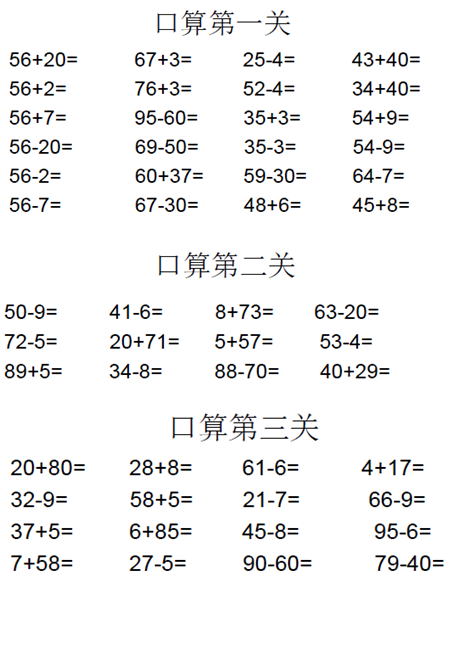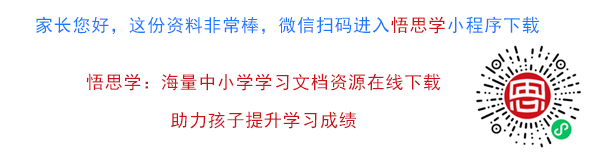56+20= 67+3= 25-4= 43+40=
56+2= 76+3= 52-4= 34+40=
56+7= 95-60= 35+3= 54+9=
56-20= 69-50= 35-3= 54-9=
56-2= 60+37= 59-30= 64-7=

【PDF文档58页】小学一年级苏教版一年级数学下册期末复习（附答案）A4电子版资料_可直接打印_会员免费下载##### 评论信息